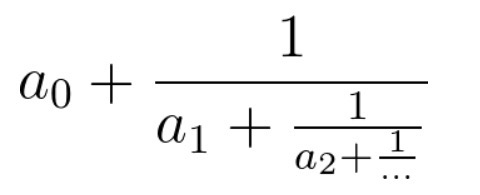2. 分式化简¶

1、题目描述¶1. cont[i] >= 0
2. 1 <= cont的长度 <= 10
3. cont最后一个元素不等于0
4. 答案的n, m的取值都能被32位int整型存下（即不超过2 ^ 31 - 1）。

2、解题思路¶

• 从后面向前计算，每次取一个值，加上后面数值的倒数
import fractions

class Solution:
def fraction(self, cont: List[int]) -> List[int]:
res = fractions.Fraction(cont.pop(), 1)
while cont:
res = cont.pop() + 1 / res

return [res.numerator, res.denominator]